# DC Motor Speed Control

Energy is of utmost importance in the contemporary world which runs at a very fast pace. DC motors play a vital role in converting electrical energy into equivalent mechanical energy. In the mechanical system, speed varies with each and every task, and thus controlling the motor speed is essential.

## DC Motor

An Electric motor is a machine that converts electrical energy into mechanical energy. Its action is based on the principle that when a current-carrying conductor is placed in a magnetic field. It experiences a mechanical force whose direction is given by Fleming’s Left-hand Rule and magnitude is given by F = BIL Newton.

Also, at times we get we need to convert the alternating components to the dc component. The two ways of doing this are half-wave rectifier and the full-wave bridge rectifier.

## Controlling Factors of DC Motor Speed Control

The equation governing the speed of a motor is given by the relation

N = K ((V – Ia*Ra)/Φ)   r.p.s.

Where Ra = armature circuit resistance

It is obvious that the speed can be controlled by varying

1. flux/pole, Φ(Flux Control)
2. Resistance Ra of armature circuit (Rheostatic Control) and
3. Applied voltage V (Voltage Control).

These methods are applied to shunt, compound and series motors will be discussed below.

## Speed Control of DC Shunt Motors

### Variation of Flux or Flux Control Method

From the above relation, we can see that speed is inversely proportional to the flux per pole. Therefore, by decreasing the flux, the speed can be increased and vice versa. Hence the name field or flux control method. The flux of a d.c. the motor can be changed by changing the shunt current with the help of the shunt field rheostat. Since shunt current(Ish )is relatively small, thus shunt field resistance has to carry only a small current, which means I2R loss is small, so that rheostat is small in size.Flux Control method of DC Motor Speed Control

This method is, therefore, very efficient. In non-interpolar machines, the speed can be increased by this method in the ratio 2:1. Any further weakening of flux adversely affects the communication and hence puts a limit to the maximum speed obtainable with this method. In machines fitted with interpoles, a ratio of maximum to a minimum speed of 6: 1 is fairly common.

### Armature or Rheostatic Control Method

This method is used when speeds below the no-load speed are required. As the supply voltage is normally constant, the voltage across the armature is varied by inserting a variable rheostat or resistance (called controller resistance) in series with the armature circuitous shown in the figure. As controller resistance is increased, the potential difference across the armature. From the speed/armature current characteristics, it is seen that the greater the resistance in the armature circuit, the greater is the fall in the speed.Armature Control Method of DC Motor Speed Control

Let,

Ia1 = armature current in the first case

Ia2 = armature resistance in the second case

N1, N2 = corresponding speeds, V = supply voltage

Let some controller resistance of value R be added to the armature circuit resistance so that its value becomes (R + Ra) = Rr

Also, N is directly proportional to the back emf (Eb1)

Therefore, N2/N1= Eb2/Eb1

Considering the no-load speed, we have N/No= (V – Ia*Rt)/ (V – Iao*Ra)

Neglecting Ia0 Ra with respect to V, we get

N = No(1- ((Ia*Rt)/V))

It is seen that for a given resistance Rt, the speed is a linear function of armature current Ia as shown in fig.

The load current for which the speed would be zero is found by putting N = 0 in the above relation.

Therefore, 0 = No(1-((Ia*Rt)/V))

###### thus, Ia = V/Rt

This is the maximum current and is known as a stalling current.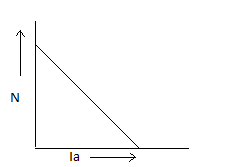Speed versus Armature Current

As shown in fig, this method is very wasteful, expensive, and unsuitable for rapidly changing loads because, for a given value of Rt, speed will change with load. A more stable operation can be obtained by using the divertor across the armature in addition to armature control resistance fig. Now, the changes in the armature current (due to changes in the load torque) will not be so effective in changing the potential difference across the armature (and hence the armature speed).## Voltage Control Method of DC Motor Speed Control

### Multiple Voltage Control

In this method, the shunt field of the motor is connected permanently to a fixed exciting voltage, but the armature is supplied with different voltages by connecting it across one of the several different voltages by means of suitable switchgear. The armature speed will be approximately proportional to these different voltages. The intermediate speeds can be obtained by adjusting the shunt field regulator. The method is not much used, however.

### Ward-Leonard System

This system is used where an unusually wide (up to 10:1) and very sensitive speed control is required as for colliery winders, electric excavators, elevators, and the main drives in steel mills and blooming and paper mills. The arrangement is illustrated in fig.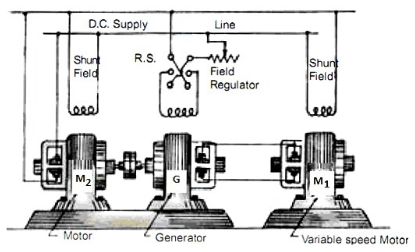Ward-Leonard System of DC Motor Speed Control

M1 is the main motor whose speed control is required. The field of this motor is permanently connected across the d.c. supply lines. Therefore, by applying a variable voltage across its armature, any desired speed can be obtained. This variable voltage is supplied by a motor-generator set which consists of either a d.c. or an a.c. motor M, directly coupled to generator G.

The motor M2 runs at an approximately constant speed. The output voltage of G is directly fed to the main motor M1. The voltage of the generator can be varied from zero up to its maximum value by means of its field regulator. By reversing the direction of the field current of G by means of the reversing switch RS, the generated voltage can be reversed and hence the direction of rotation of M1. It should be remembered that the motor-generator set always runs in the same direction.

The chief disadvantage of this system is its low overall efficiency, especially at light loads. But as said earlier, it has the outstanding merit of giving wide speed control from maximum in one direction through zero to the maximum in the opposite direction and of giving a smooth acceleration.

## Speed Control of DC Series Motors

### Flux Control Method

Variations in the flux of the series motor can be brought about in any one of the following ways:

#### a) Field Divertors

The series windings are shunted by a variable resistance known as field divertor, see fig. Any desired amount of current can be passed through the divertor by adjusting its resistance. Hence the flux can be decreased and consequently, the speed of the motor increases.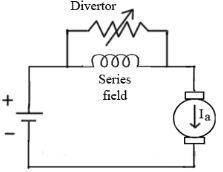Field Divertor for DC Motor Speed Control

#### b) Armature Divertors

A divertor across the armature can be used for giving speeds lower than the normal speed, see fig. For a given constant load torque, if Ia is reduced due to an armature divertor, the flux must increase.Armature Divertor for DC Motor Speed Control

This results in an increase in current taken from the supply (which increases the flux and a fall in speed). The variation in speed can be controlled by varying the divertor resistance.

#### c) Trapped Field Control Field

This method is often used in electric traction. See the figure below. The number of series field turns in the circuit can be changed at will as shown. With a full field, the motor runs at its maximum speed which can be raised in steps by cutting out some of the series turns.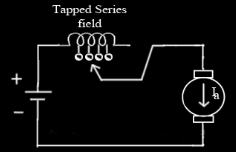Trapped field control for DC Motor Speed Control

#### d) Paralleling Field Coils

In this method, used for fan motors, several speeds can be obtained by regrouping the field coils as shown in fig. It is, therefore, seen that for a 4-pole motor, three speeds can be obtained easily.Paralleling field coils

### Variable Resistance in Series with Motor

By increasing the resistance in series with the armature, (see the fig) the voltage applied across the armature terminals can be decreased. With reduced voltage across the armature, the speed reduces. However, it will be noted that since full motor current passes through this resistance, there is a considerable loss of power in it.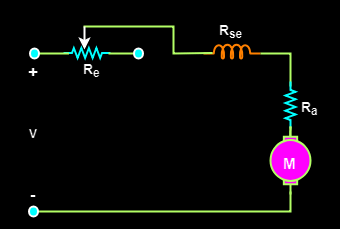Variable resistance in series for DC Motor Speed Control

## Merits and Demerits of Rheostatic DC Motor Speed Control

• Speed changes with every change in load, because speed variations depend not only on controlling resistance but on load current also. This double dependence makes it impossible to keep the speed sensibly constant on rapidly changing loads.
• A large amount of power gets waste in the controller resistance. Loss of power is directly proportional to the reduction in speed. Hence efficiency decreases.
• Maximum power developed diminishes in the same ratio as speed.
• It needs an expensive arrangement for the dissipation of heat produced in the controller resistance.
• It gives speed below the normal, not above it because the armature voltage can be decreased (not increased) by the controller resistance.

This method is, therefore, employed when low speeds are required for a short period only and that too occasionally as in printing machines and for cranes and hoists where the motor is continually started and stopped.

## Advantages of Field Control of DC Motor Speed Control

•  This method is economical, more efficient, and convenient though it can give speeds above (not below) the normal speed.
•  The only limitation of this method is that commutation becomes unsatisfactory because the effect of armature reaction is greater on a weaker field.

It should, however, be noted that by combining the two methods, speeds above and below the normal may be obtained. Therefore we achieve the desired DC Motor speed control.

•
•
•
•
•
•